# SAT Math Multiple Choice Question 884: Answer and Explanation

### Test Information

Question: 884

14.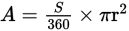The area of a sector of a circle is given by the formula above, where S is the angle measure in degrees of the sector and r is the radius of the circle. Which of the following gives r in terms of A and S?

• A.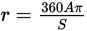• B.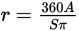• C.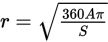• D.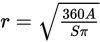Explanation:

D

Difficulty: Medium

Category: Passport to Advanced Math / Exponents

Strategic Advice: Don't let all the variables in a question like this intimidate you. Pretend they're simple numbers and use inverse operations to solve for the desired variable.

Getting to the Answer: To solve the equation for r, start by multiplying both sides of the equation by the only number that's in the denominator on the right-hand side (360). Then, divide by everything that r is being multiplied by (Sπ):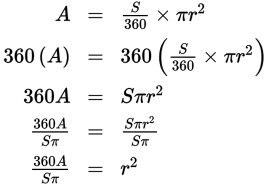Don't answer too quickly! The correct answer is not B. You're asked for r (not r2), so take the square root of both sides of the equation to find that, which is (D).